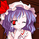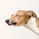2857 obejrzeń
2857
Combines SuperTrend with Stochastic Oscilator to provide entry and exit signals.

Offers 3 types of entry signals with an option to color ideal entry bars.

- Entry after SuperTrend flip confirmation.
- Entry at over bought/oversold levels respective to the trend.
- Entry at the 50% stochastic level following trend direction.

Offers two types of exit signals.
- SuperTrend as a trailing stop.
- Exit at over bought/oversold levels respective to the trend.

- ChopZone coloring of SuperTrend dots.
- Coloring of ideal bars showing when stochastic %k crosses %d.

Best time-frame is 1h, however it may be used on any time-frame.
Back-testing on the GBP/USD chart showed an average of 9% monthly gains with few losses.

Remember to trade responsibly and manage your risk. The system is not a guarantee of gains and is subject to the whim of fundamentals. Draw boxes or trend-lines to show
your area's of risk/reward. A box of 0.1% can help to maximize gains and minimize losses.

If this system has earned you profits, please donate.
- PayPal: ma.spencer@gmx.com
- Bitcoin: 1foxypuyuoNp5n1LNCCCCmjZ4RAXntQ8X

Preview of usage (v1.0) - Back-test (v1.0) -
```// Coded by: Matthew Spencer
// If you like this indicator and/or it has helped you to earn money, please donate.
// Paypal: ma.spencer@gmx.com
// Bitcoin: 1foxypuyuoNp5n1LNCCCCmjZ4RAXntQ8X
// "If I die in the land of the dead, will I be sent to the land of the dead again?"

study("UberTrend v1.1", overlay = true) // Declare the study

// SuperTrend

// Define Inputs
separator1 = input(title="---", type=string, defval="SuperTrend")
Multiplier=input(3, type="float", minval=1,maxval = 100)
Periods=input(10, minval=1,maxval = 100)

// Calculate ATR for trend
Up=hl2-(Multiplier*atr(Periods))
Dn=hl2+(Multiplier*atr(Periods))

// Calculate trend
TrendUp=close>TrendUp? max(Up,TrendUp) : Up
TrendDown=close<TrendDown? min(Dn,TrendDown) : Dn
Trend = close > TrendDown ? 1: close< TrendUp? -1: nz(Trend,1)
Tsl = Trend==1? TrendUp: TrendDown

linecolor = Trend == 1 ? green : red // Flip colors

lineToggle = input(title="Show Line", type=bool, defval=true)
dotsToggle = input(title="Show Dots", type=bool, defval=true)
chopToggle = input(title="ChopZone Dots", type=bool, defval=false)

// End of SuperTrend

// Stochastic %k

// Define inputs
separator2 = input(title="---", type=string, defval="Stochastic")
kPeriod = input(7, minval=1, type=integer)
kMin = input(30, minval=1, type=float) // OverSold
kMax = input(70, minval=1, type=float) // OverBought

// Toggles
separator3 = input(title="---", type=string, defval="Signals")
longToggle = input(title="Long Entry", type=bool, defval=true)
longAltToggle = input(title="Long Alt Entry", type=bool, defval=true)
longExitToggle = input(title="Long Exit", type=bool, defval=true)
shortToggle = input(title="Shot Entry", type=bool, defval=true)
shortAltToggle = input(title="Short Alt Entry", type=bool, defval=true)
shortExitToggle = input(title="Shot Exit", type=bool, defval=true)

// Calculate Stochastic
kHigh = highest(high, kPeriod)
kLow = lowest(low, kPeriod)
k1 = close -kLow
k2 = kHigh - kLow
k = sma(k1/k2 * 100, 3)
d = sma(k, 3)
// End of Stochastic %k

// ChopZone
colorTurquoise = #34dddd
colorDarkGreen = #006400
colorPaleGreen = #98fb98
colorLime = lime
colorDarkRed = #8B0000
colorRed = red
colorOrange = orange
colorLightOrange = #ffc04c
colorYellow = yellow

source = close
avg = hlc3

pi = atan(1) * 4
periods = 30
highestHigh = highest(periods)
lowestLow = lowest(periods)
range = 25 / (highestHigh - lowestLow) * lowestLow

ema34 = ema(source, 34)
x1_ema34 = 0
x2_ema34 = 1
y1_ema34 = 0
y2_ema34 = (ema34 - ema34) / avg * range
c_ema34 = sqrt((x2_ema34 - x1_ema34)*(x2_ema34 - x1_ema34) + (y2_ema34 - y1_ema34)*(y2_ema34 - y1_ema34))
emaAngle_1 = round(180 * acos((x2_ema34 - x1_ema34)/c_ema34) / pi)
emaAngle = iff(y2_ema34 > 0, - emaAngle_1, emaAngle_1)

chopZoneColor = emaAngle >= 5 ? colorTurquoise : emaAngle < 5 and emaAngle >= 3.57 ? colorDarkGreen : emaAngle < 3.57 and emaAngle >= 2.14 ? colorPaleGreen : emaAngle < 2.14 and emaAngle >= .71 ? colorLime : emaAngle <= -1 * 5 ? colorDarkRed : emaAngle > -1 * 5 and emaAngle <= -1 * 3.57 ? colorRed : emaAngle > -1 * 3.57 and emaAngle <= -1 * 2.14 ? colorOrange : emaAngle > -1 * 2.14 and emaAngle <= -1 * .71 ? colorLightOrange : colorYellow
// End of ChopZone

// Plot Super Trend
plot(lineToggle ? Tsl :na, color = linecolor , style = line , linewidth = 2,title = "SuperTrend")    // Plot Line
plot(dotsToggle ? Tsl :na, color = chopToggle ? chopZoneColor : linecolor , style = circles , linewidth = 4,title = "SuperTrend", editable = false)// Plot Dots

// Plot entries
plotshape(cross(close,Tsl) and close>Tsl , "SuperTrend Long", shape.triangleup,location.belowbar,teal,0,1) // Super Trend Long
plotshape(cross(Tsl,close) and close<Tsl , "SuperTrend Short", shape.triangledown , location.abovebar, teal,0,1) // Super Trend Short
plotshape(longToggle ? (k <= kMin and Trend == 1 ? Trend : na) : na, "Long Entry", shape.labelup,location.belowbar,navy,0,0, "", black) // Stochastic Long Entry
plotshape(longAltToggle ? (rising(k,1) and cross(k, 50) and Trend == 1 ? Trend : na) : na, "Long Alt Entry", shape.cross,location.belowbar,olive,0,0, "", black) // Stochastic Long Entry
plotshape(longExitToggle ? (k >= kMax and Trend == 1 ? Trend : na) : na, "Long Exit", shape.xcross,location.abovebar,red,0,0, "", black) // Stochastic Long Exit
plotshape(shortToggle ? (k >= kMax and Trend == -1 ? Trend : na) : na, "Short Entry", shape.labeldown , location.abovebar, navy,0,0, "", black) // Stochastic Short Entry
plotshape(shortAltToggle ? (falling(k,1) and cross(k, 50) and Trend == -1 ? Trend : na) : na, "Short Alt Entry", shape.cross , location.abovebar, olive,0,0, "", black) // Stochastic Short Entry
plotshape(shortExitToggle ? (k <= kMin and Trend == -1 ? Trend : na) : na, "Short Exit", shape.xcross , location.belowbar, red,0,0, "", black) // Stochastic Short Exit

// Bar Color Toggle
barToggle = input(title="Color Ideal Bars", type=bool, defval=true)
// Color Ideal Long
barcolor(barToggle ? (cross(k,d) and k <= kMin and Trend == 1 ? lime : na) : na, 0 , false)
barcolor(barToggle ? (cross(k,d) and k >= kMax and Trend == -1 ? lime : na) : na, 0 , false)
// Color Ideal Short
barcolor(barToggle ? (cross(k,d) and k >= kMax and Trend == 1 ? orange : na) : na, 0 , false)
barcolor(barToggle ? (cross(k,d) and k <= kMin and Trend == -1 ? orange : na) : na, 0 , false) ```kris00l
@karabogerald @kris001 Not sure what would be causing that issue as Line 9 is a comment. Unless it isn't counting comments in which case Line 23 would be the issue. It has been 10 months since I had written the script and I know that TradingView has made many changes to Pine Script since then. Perhaps something broke as it changed. I have college finals this and next week so I may not be able to fix it right away, but will look into it and maybe rewrite it entirely when I have the free time to do so.
Odpowiedźgenefy
The issue is a syntax error. If you change line 13:

From
Multiplier=input(3, type="float", minval=1,maxval = 100)

To
Multiplier=input(3, type=float, minval=1,maxval = 100)

It should be fine. Working for me anyway.
Odpowiedźgenefy
@genefy, I've been planning to remake it eventually, just really busy trying to get through school and find employment. Script is for a very old version of Pine and needs to be rewritten.
Odpowiedźgenefy
@genefy, I was thinking it'd be more accurate to use William's %R when I finally have the time to remake it. I gotta learn the new Pine script now that strategies are a thing.
Odpowiedź